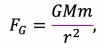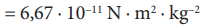# Newton´s law of universal gravitation

Newton's law of gravitation describes the gravitational attraction of two objects with masses ? and ?, which can be homogeneous spheres2 (mass is evenly distributed throughout the volume of the object), or the dimensions of the objects are negligible compared to the mutual distance. Then we idealize objects with material points, characterized only by their mass.

The relation for the magnitude of the gravitational force between two objects is given by the formula:whereis gravitational constant, ? and m are masses of objects, r is distance between objects, alternatively between centres of spherical objects.

Note: For more complex objects, the gravitational force cannot be calculated by simply adding masses and distances into Newton's law. It is necessary to use more advanced mathematics, which students will encounter only in the 4th year of secondary school when teaching differential and integral calculus.

filter_none